• 查找函数 1. vlookup函数 vlookup(lookup_value,table_array,col_index_num,[range_lookup]) lookup_value表示要查找的对象 table_array表示查找的表格区域 col_index_num表示要查找的数据在table_array区域中处于...
一. 查找函数
1. vlookup函数
vlookup(lookup_value,table_array,col_index_num,[range_lookup])
lookup_value表示要查找的对象table_array表示查找的表格区域col_index_num表示要查找的数据在table_array区域中处于第几列的列号range_lookup表示查找类型，其中1表示近似匹配，0表示精确匹配，一般我们用精确匹配的情况较多
A1:B7表示要在其中查找的区间，也就是公式中的table_array D1到D4中表示我们要查找的对象，也就是公式中的lookup_value 现在我们要查找D1到D4分别对应的数值。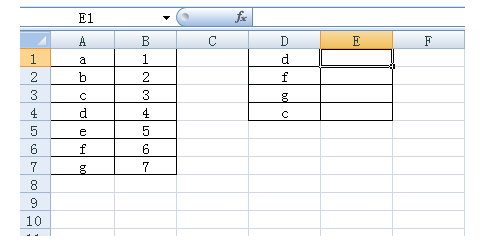点击公式菜单栏——查找与引用——vlookup 在出现的vlookup界面中第一栏选中D1 第二栏选中A1：D7 第三栏输入2(表示引用的是A1:D7区域的第2列) 第四栏输入0(精确匹配)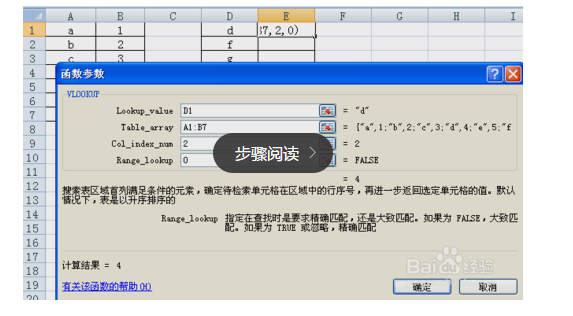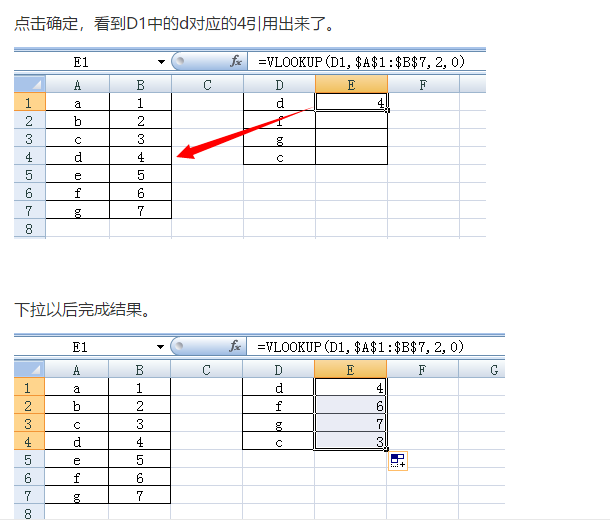与lookup和hlookup的区别：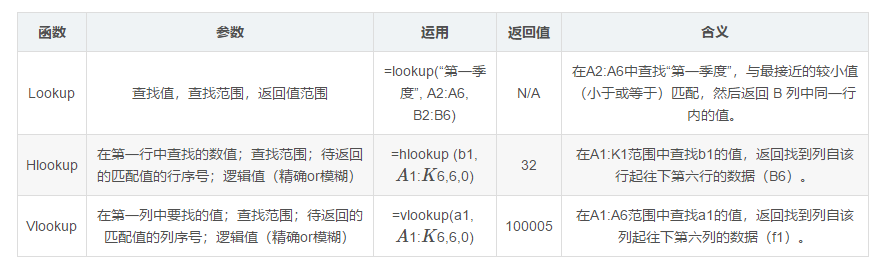HLOOKUP函数和VLOOKUP函数的差异是查询的方向不一样。 VLOOKUP函数是按列方向（向右）查找，HLOOKUP函数是按行方向（向下）查找。
2. match和index
MATCH(lookup-value,lookup-array,match-type) (返回指定内容所在的位置）
lookup-value:表示要在区域或数组中查找的值，可以是直接输入的数组或单元格引用lookup-array:表示可能包含所要查找的数值的连续单元格区域，应为数组或数组引用match-type:表示查找方式，用于指定精确查找（查找区域无序排列）或模糊查找（查找区域升序排列）。取值为-1、1、0 。其中0为精确查找INDEX(array,row-num,column-num) （返回制定位置中的内容）array:要返回值的单元格区域或数组row-num:返回值所在的行号column-num:返回值所在的列号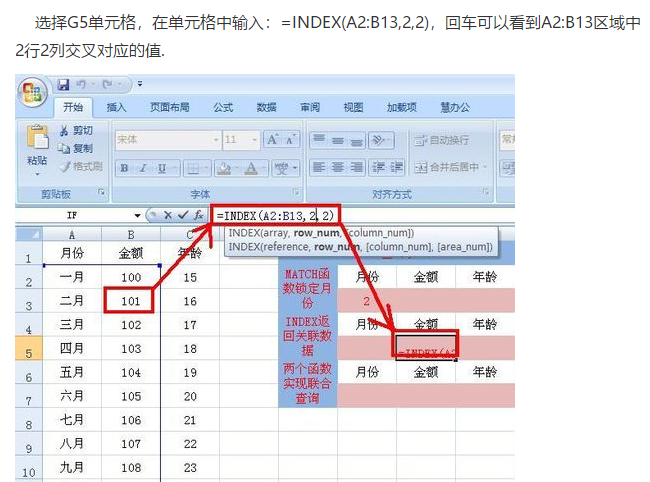用match和index函数实现vlookup功能 选择G7单元格，在单元格中输入：=INDEX(B2:B13,MATCH(F7,A2:A13,0))，用MATCH函数返回F7单元格中的之所对应的行，再利用ATCH函数返回的行号用INDEX函数查询出月份对应的金额。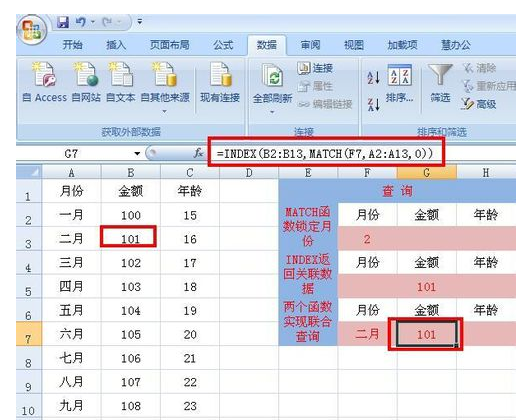3. 双条件查找
3.1 vlookup函数。
如下图所示，根据班级和姓名该如何查找成绩呢？先放公式：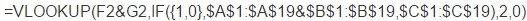此公式为数组函数，输完公式后按下ctrl+shift+enter键计算结果。这个公式咋一看看挺长，不过不要担心，现在就为你详细地解释这个公式到底是什么意思。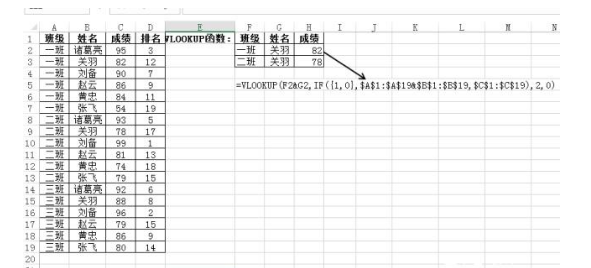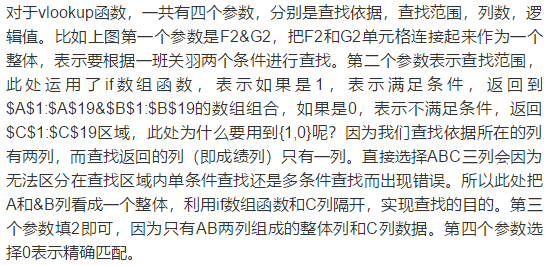3.2 index—match函数
如果实现与上面vlookup函数同样的查找结果运用index—match函数的公式为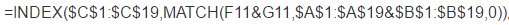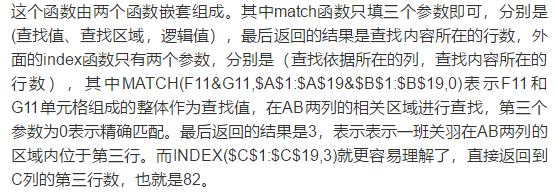二. 作业
1. 用vlookup函数 查找以下公司的 companyId
| companyFullName | |:----| | 上海云贝网络科技有限公司 | | 携程计算机技术（上海）有限公司 | | 浙江康健绿线网络技术有限公司 | | 久亿财富（北京）投资有限公司 | | 杭州木瓜科技有限公司 | | 思特沃克软件技术（成都）有限公司 | | 北京金山云网络技术有限公司 |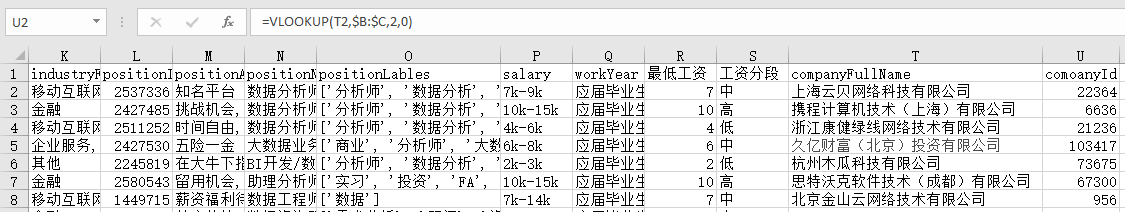2. 用match和index函数实现第一题的功能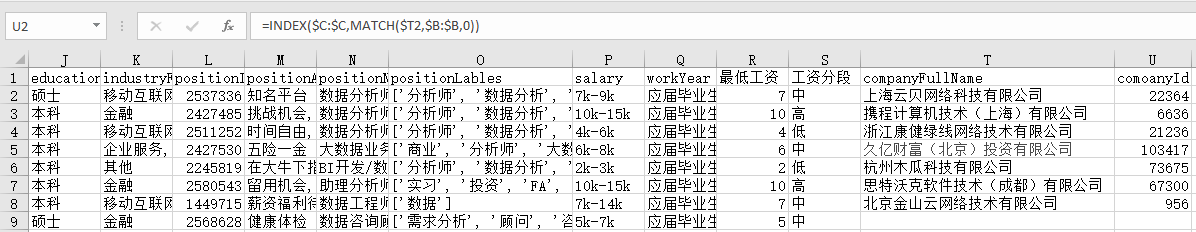3. 用match和index函数查找以下id对应的公司名称
注意id是横向排列的| companyId | 127200 | 151079 | 22225 | |----|----|----|----|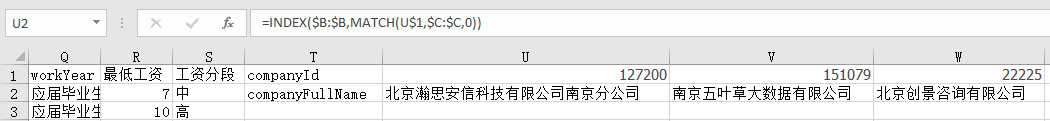4. 根据companyId和postionId两个条件查找对应的工资水平
| companyId | positionId | salary | | 62 | 938038 | | 1575 | 1157620 | | 157392 | 2574696 |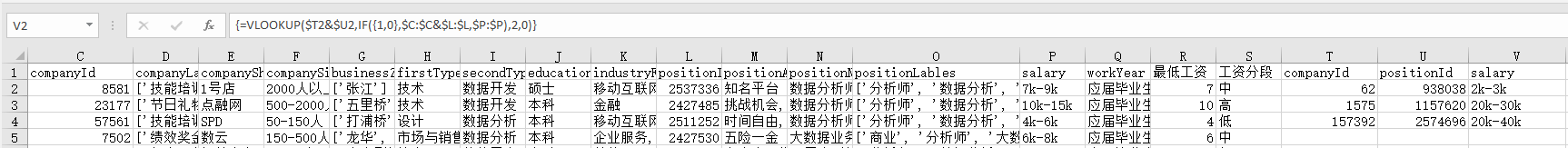注意：在输入公式后，不是按Enter，而是按Ctrl+Shift+Enter。CTRL+SHIFT+ENTER在Excel中是用于计算数组公式使用的。数组公式运用前是要以数组的形式嵌入公式，要选定数组区间，这样才能执行数组计算，得出的结果才是正确的。（不要自己在两边输入{}，否则Excel认为输入的是一个正文标签。）
请思考，是否会存在相同的公司id和职位di对应多种工资水平，如果有请查找出来。 并思考，如果存在多种的情况，目前的公式还能不能用？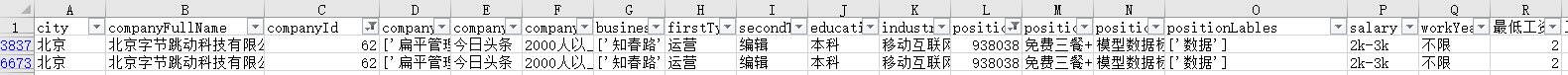很明显是存在的，目前的公式还能用，但是只返回查找到的第一个值，假如第一行salary是2k-4k，则最终返回结果就是2k-4k。
展开全文• Vlookup函数> 用途：用通俗的话就是根据现有条件查找到符合条件的值再黏贴过来。 返回与查询值同行的返回列值。 语法：Vlookup（查询值，查询范围，返回列数，匹配方式) ①第1个参数：选中查找范围里的第一列...
<Vlookup函数>
用途：返回与查询值同行的返回列值。
用通俗的话就是根据现有条件查找到符合条件的值再黏贴过来。
语法：Vlookup（查询值，查询范围，返回列数，匹配方式)
①第1个参数：查找范围第一列的查找值。选中查找范围里的第一列的值必须是要查找的值。
②第2个参数：查找范围，可以理解为数据表。使用F4按钮灵活转换绝对引用和相对引用，使查找范围全部选中。
③第3个参数：返回值的列号。指定要返回查询区域中第几列的值。
③第4个参数：匹配方式是否为模糊匹配。模糊匹配为1，精确匹配为0，默认为模糊匹配。

用SQL来理解就容易很多了：
select ③
from ②
where ②的第一列=①
and 匹配方式=④是否模糊匹配（模糊匹配=1，精确匹配=0，默认是模糊匹配）

vlookup函数的实战用法
Vlookup函数用法1：常规查询
【案例1】根据第一张表，查询哪位销售员下了订单编号为“0001”的订单。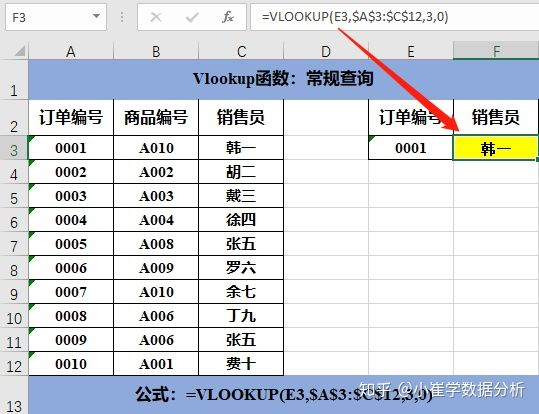公式：=VLOOKUP(E3,$A$3:$C$12,3,0)
解读：这是vlookup函数最常规的一种用法。
Vlookup函数用法2：数据分组
【案例2】根据第二张表的阈值及分组，匹配出与销售额所在范围的分组情况。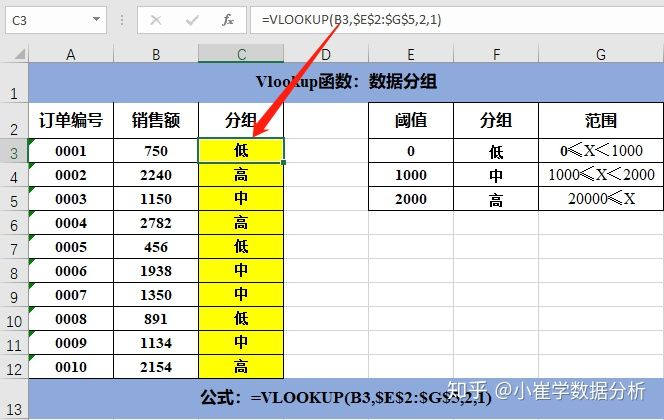公式：=VLOOKUP(B3,$E$2:$G$5,2,1)
解读：vlookup函数的第四个参数为1或省略时，则表示此时的vlookup函数使用模糊匹配。返回的值为小于查询值的最大值。在使用模糊匹配时，查询范围的首列的阈值必须从小到大排序。
Vlookup函数用法3：反向查询
【案例3】根据第一张表，查询出销售员徐四销售出去的商品编号。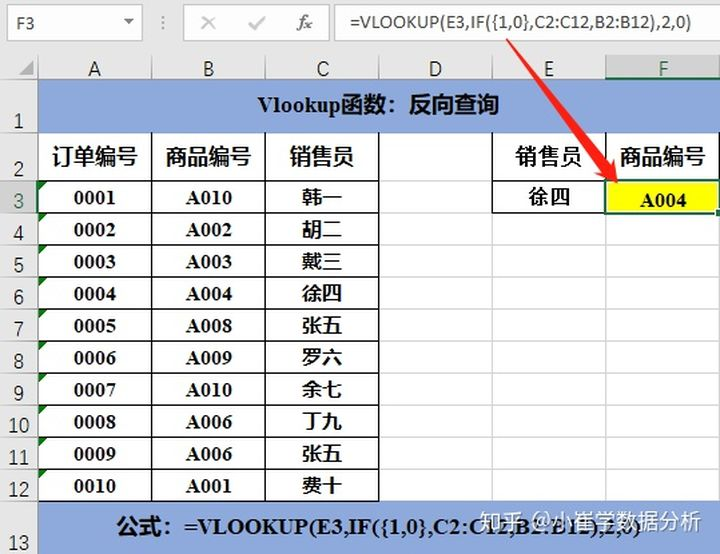公式：=VLOOKUP(E3,IF({1,0},C2:C12,B2:B12),2,0)
解读：if函数使用方式：if({1,0},查找关键字所在列,返回值所在列)
这个if函数使用起来可以把查找关键字所在列和返回值所在列这两列的顺序进行反向调整。
Vlookup函数用法4：模糊匹配
【案例4】根据第一张表，查询出姓“丁”的销售员姓名和销售额。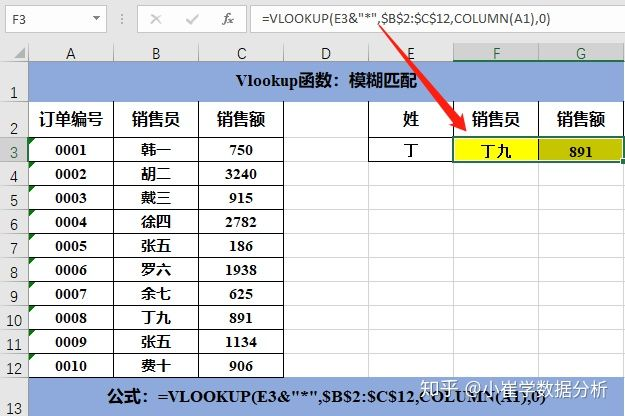公式：=VLOOKUP(E3&"*",$B$2:$C$12,COLUMN(A1),0)
解读：返回值所在列使用通配符 * 可以替代任意多个字符；
使用column函数返回指定单元格的列数；
在单元格F9中使用该函数可以返回姓“丁”的销售员的全名，往右拉单元格可以得到该销售员的销售额。
Vlookup函数用法5：多条件查询
【案例5】根据第一张表，查询产品为“0001”，型号为“A001”的产品的产地。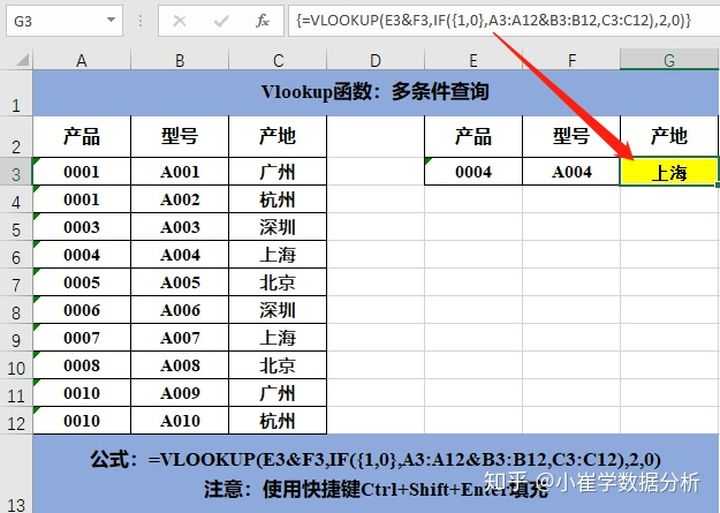公式：=VLOOKUP(E3&F3,IF({1,0},A3:A12&B3:B12,C3:C12),2,0)
解读：if函数使用方式：if({1,0},查找关键字所在列,返回值所在列)
当我们需要查询匹配多个条件时，需要用连接符号 & 把多个查询条件连接在一起，并且用连接符号 & 把多个查询范围连接在一起。
Vlookup函数用法6：多条件反向查询
【案例6】根据第一张表，查询产品为“0003”型号为“A003”的产品的产地。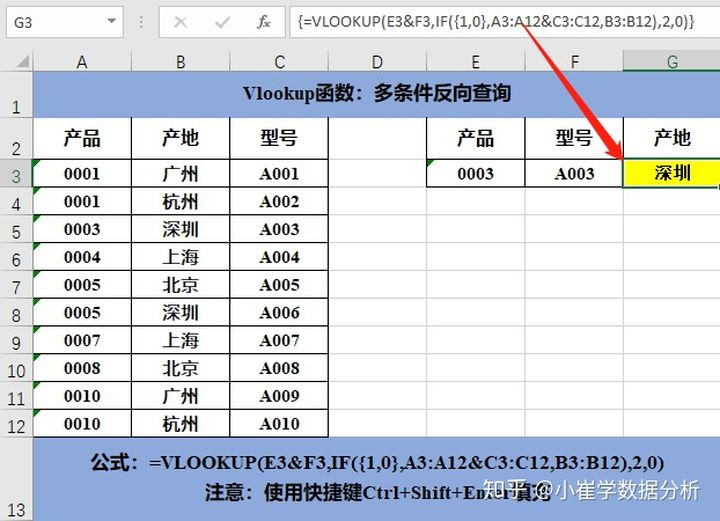公式：=VLOOKUP(E3&F3,IF({1,0},A3:A12&C3:C12,B3:B12),2,0)
解读：if函数使用方式：if({1,0},查找关键字所在列,返回值所在列)
当我们需要查询匹配多个条件时，需要用连接符号 & 把多个查询条件连接在一起，并且用连接符号 & 把多个查询范围连接在一起。
注意：在使用数组的公式后不能只按Enter，这时要使用快捷键Ctrl+Shift+Enter填充。
Vlookup函数用法7：批量查询
【案例7】根据第一张表，查询一系列订单编号的商品编号，销售额，销售员。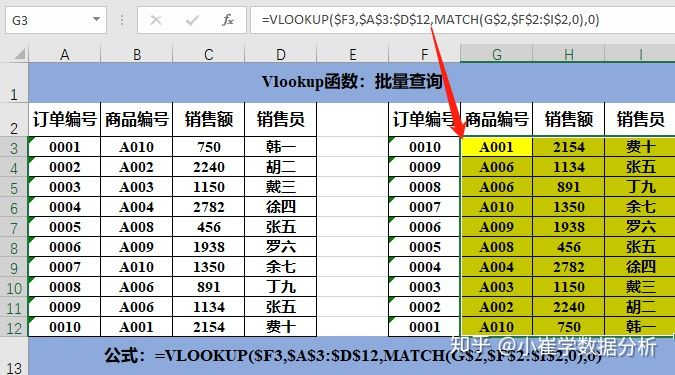公式：=VLOOKUP($F3,$A$3:$D$12,MATCH(G$2,$F$2:$I$2,0),0)
解读：使用match函数可以返回指定值在数组中的位置，在vlookup函数第三个参数的查询列使用match函数，可以返回查询值对应的列数。
在每个参数里合理使用相对引用，绝对引用和混合引用，可以一次性填充进去。
Vlookup函数用法8：交叉查询
【案例8】根据第一张表，查询销售员张五和丁九第三季度的销售额。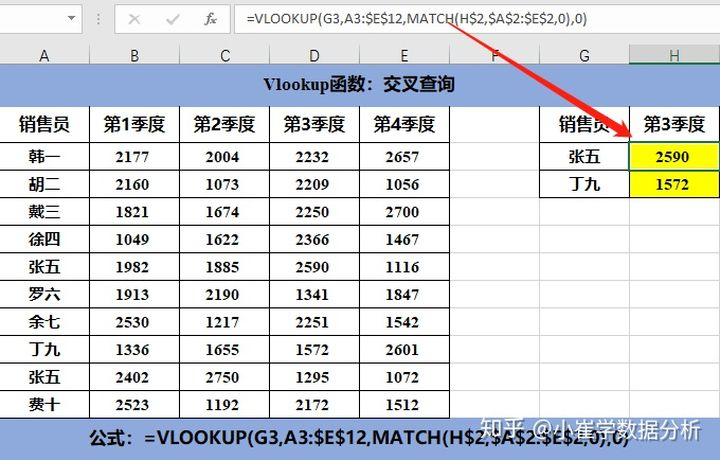公式：=VLOOKUP(G3,A3:$E$12,MATCH(H$2,$A$2:$E$2,0),0) 解读：使用match函数可以返回指定值在数组中的位置，在vlookup函数第三个参数的查询列使用match函数，可以返回查询值对应的列数。 Vlookup函数用法9：一对多查询 【案例9】根据第一张表，查询销售员韩一所有的订单编号。 ①一对多查询（纵向排列） 第一种方法：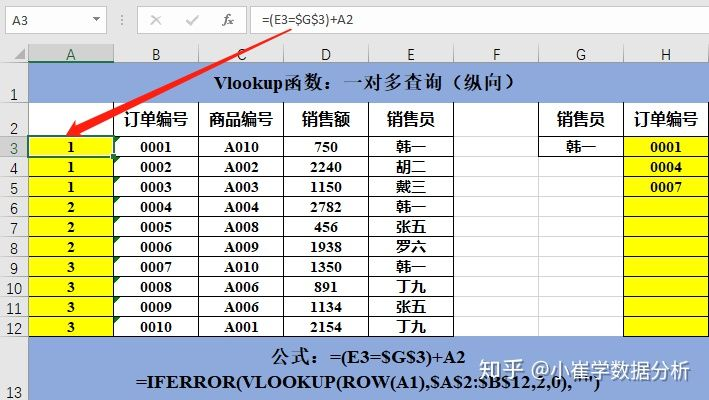公式：=(E3=$G$3)+A2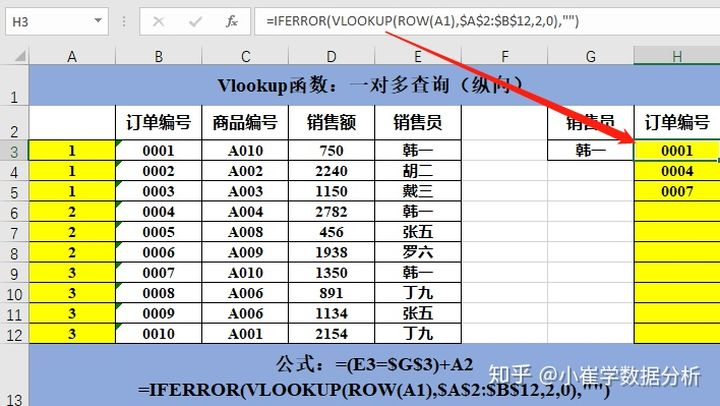公式：=IFERROR(VLOOKUP(ROW(A1),$A$2:$B$12,2,0),"") 解读：第一步：先添加辅助列。 在单元格A3中输入公式=(E3=$G$3)+A2，可以计算出E列中销售员“韩一”每出现一次就会在相应的A列加1。 第二步：结合使用iferror函数，row函数和vlookup函数匹配得到第一个符合查询条件的数据，然后下拉返回所有符合查询条件的数据。 第二种方法：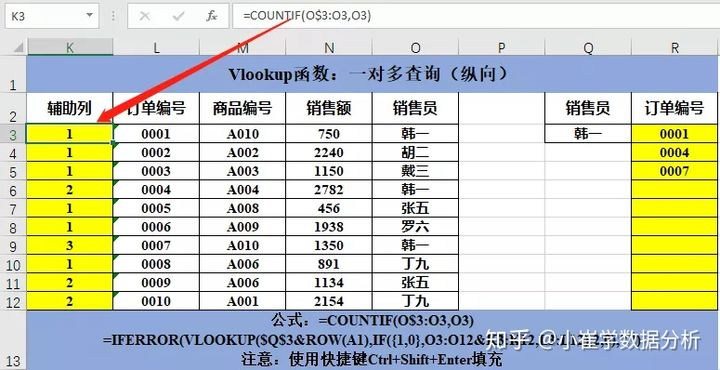公式：=COUNTIF(O$3:O3,O3)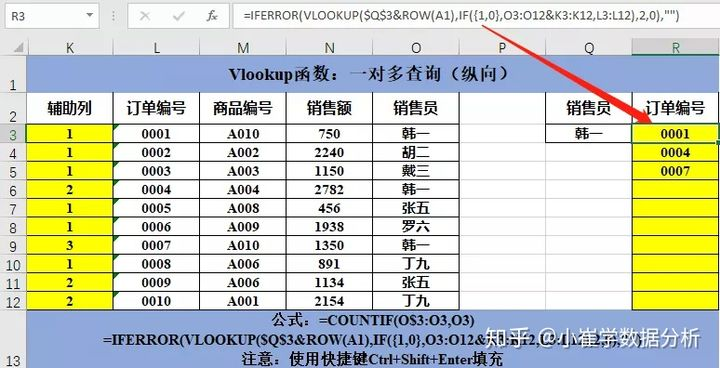公式：=IFERROR(VLOOKUP($Q$3&ROW(A1),IF({1,0},O3:O12&K3:K12,L3:L12),2,0),"")
解读：第一步：先添加辅助列。
使用countif函数统计了销售员“韩一”在对应区域出现了多少次。
第二步：结合使用iferror函数，row函数和vlookup函数，并使用快捷键Ctrl+Shift+Enter填充得到所有符合条件的值。
注意：在使用数组的公式后不能只按Enter，这时要使用快捷键Ctrl+Shift+Enter填充。
②一对多查询（横向排列）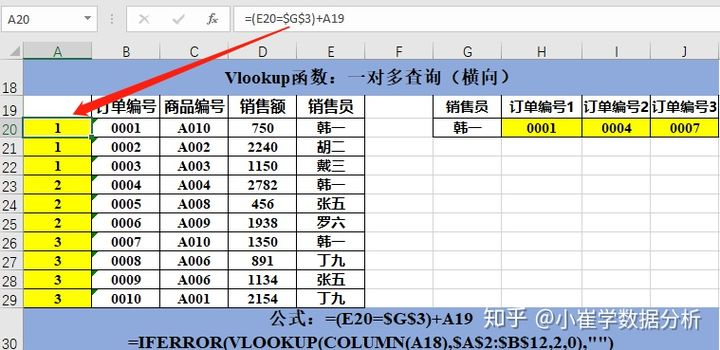公式：=(E20=$G$3)+A19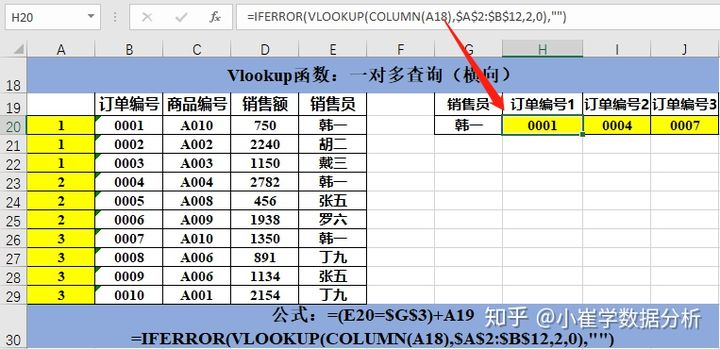公式：=IFERROR(VLOOKUP(COLUMN(A18),$A$2:$B$12,2,0),"")
解读：第一步：先添加辅助列。
在单元格A20中输入公式=(E20=$G$3)+A19，可以计算出E列中销售员“韩一”每出现一次就会在相应的A列加1。
第二步：结合使用iferror函数，row函数和vlookup函数匹配得到第一个符合查询条件的数据，然后右拉返回所有符合查询条件的数据。
Vlookup函数用法10：合并同类项
【案例10】根据第一张表，把按照组别分类的销售员姓名合并在一行。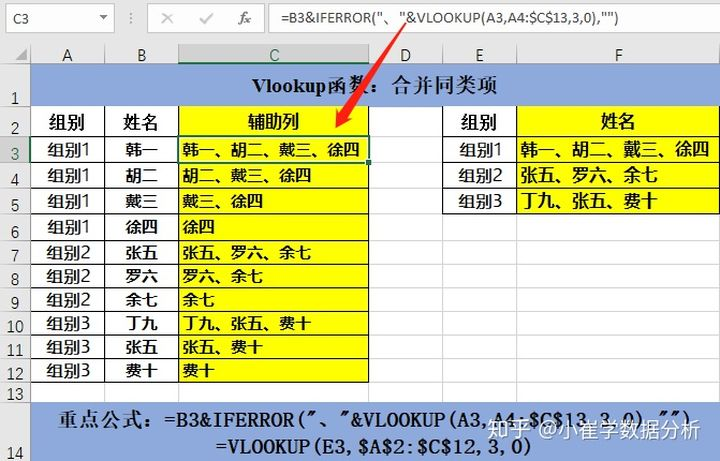重点公式：=B3&IFERROR("、"&VLOOKUP(A3,A4:$C$13,3,0),"")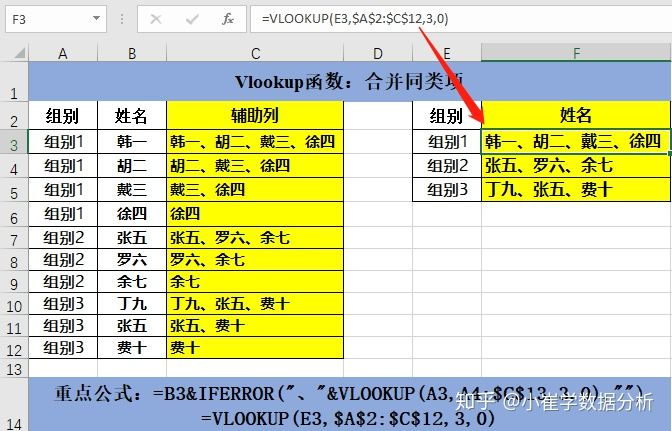公式：=VLOOKUP(E3,$A$2:$C$12,3,0)
解读：第一步：先添加辅助列。
公式=B3&IFERROR("、"&VLOOKUP(A3,A4:$C$13,3,0),"")中，由于已经使用&把第一个数据放在单元格最开始，而vlookup函数第四个参数为0时，返回第一个符合条件的数据，所以vlookup函数的查询范围必须是从下一行开始进行匹配。这样，我们就可以依次返回查询值，最终在每一个组别的第一行会返回符合条件的全部数据。
第二步：使用vlookup函数匹配进行常规查询，返回符合条件的从上往下的第一个数据。
Vlookup函数用法11：多工作表查询
【案例11】前三张表是每个组中每个销售员的订单编号，根据前三张表，查询不按照组别分组的部分销售员下的订单编号。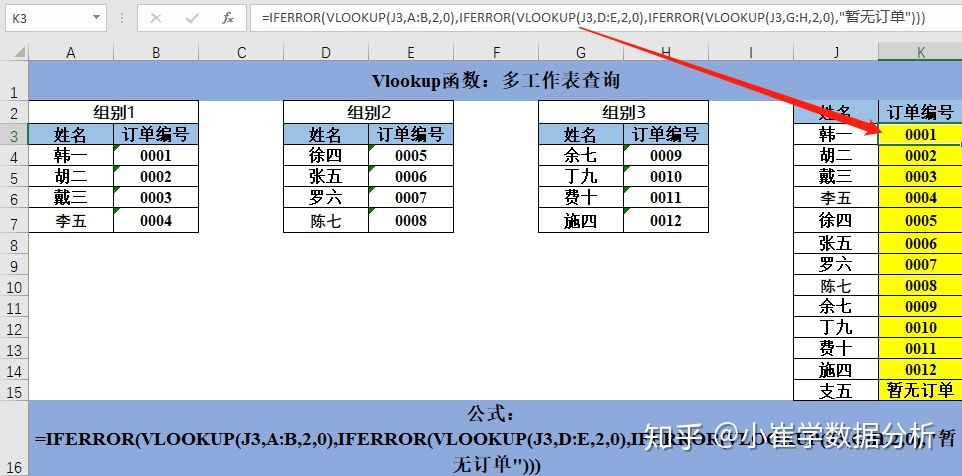公式：=IFERROR(VLOOKUP(J3,A:B,2,0),IFERROR(VLOOKUP(J3,D:E,2,0),IFERROR(VLOOKUP(J3,G:H,2,0),"暂无订单")))
解读：vlookup函数与iferror函数结合使用，可以查找匹配多张工作表或者多张工作薄。

以上为vlookup函数的11种用法。

参考链接：https://zhuanlan.zhihu.com/p/148544332

vlookup函数、hlookup函数 分别按列查找同行数据、按行查找同列数据
vlookup中的v、hlookup中的h分别代表什么单词的意思？
一般凡是这种V和H相对应的都表示垂直（vertical）与水平（horizontal）
VLOOKUP中的V，它指的是Vertical，纵向的意思，如VLOOKUP，指在指定的范围内纵向查找目标内容。
数据可以用第一行列出字段，也可以用第一列列出字段，一般用行列出，而不是用列列出，类似于数据库的表，所以vlookup函数用得比较多。


展开全文• ## 查找函数

千次阅读 2018-11-18 16:59:52
查找函数 1 VLOOKUP函数 = VLOOKUP ( 查找值 , 查找的区域 , 返回位置(列) , 查找方式 ) 精确查找 模糊查找 =VLOOKUP(值,区域,返回列,false) =VLOOKUP(值,区域,返回列,true) =...
查找函数
1 VLOOKUP函数  = VLOOKUP ( 查找值 , 查找的区域 , 返回位置(列) , 查找方式 )  精确查找 模糊查找  =VLOOKUP(值,区域,返回列,false) =VLOOKUP(值,区域,返回列,true)  =VLOOKUP(值,区域,返回列,0) =VLOOKUP(值,区域,返回列,1)  =VLOOKUP(值,区域,返回列,) =VLOOKUP(值,区域,返回列)  注意：如果查找方式是为模糊查找，那么区域一定要为升序！否则会出错。  2  = HLOOKUP ( 查找的值 ， 查找的区域 ， 返回的行号 ， 查找方式 )  其余和VIOOKUP函数一样
3 = LOOKUP ( lookup_value , lookup_vector , result_vector )  第一参数：查找的数值。可以为数字、文本、逻辑值或包含数值的名称或引用。 第二参数：为只包含一行或一列的区域。 第三参数：可以为文本、数字或逻辑 ，只包含一行或一列的区域，其大小必须与 lookup_vector 相同。 注意：Lookup_vector 的数值必须按升序排序；否则，函数 LOOKUP 不能返回正确的结果。文本不区分大小写。
4= CHOOSE ( index_num , value1)  第一参数：Index_num 必须为 1 到 29 之间的数字、或者是包含数字 1 到 29 的公式或单元格引用  第二参数：函数 CHOOSE 基于 index_num，从中选择一个数值或执行相应的操作。参数可以为数字、单元格引用、已定义的名称、公式、函数或文本。
5 = MATCH ( lookup_value , lookup_array , match_type)
第一参数：为需要在数据表中查找的数值。可以为数值（数字、文本或逻辑值）或对数字、文本或逻辑值的单元格引用。可能包含所要查找的数值的连续单元格区域。 第二参数：Lookup_array 应为数组或数组引用为数字 第三参数：为 -1、0 或 1。Match-type 指明 Microsoft Excel 如何在 lookup_array 中查找 lookup_value。
6 = INDEX ( array , row_num , column_num )  第一参数：为单元格区域或数组常量  第二参数：数组中某行的行序号，函数从该行返回数值。如果省略 row_num，则必须有 column_num。  第三参数：数组中某列的列序号，函数从该列返回数值。如果省略 column_num，则必须有 row_num。
7 =OFFSET ( reference , rows , cols , height , width) 以指定的引用为参照系，通过给定偏移量得到新的引用。返回的引用可以为一个单元格或单元格区域。并可以指定返回的行数或列数。  第一参数：偏移量参照系的引用区域； 第二参数：行偏移量。 第三参数：列偏移量； 第四参数：高度，且必须为正数； 第五参数：宽度，也必须为正数。
8返回由文本字符串指定的引用。此函数立即对引用进行计算，并显示其内容。当需要更改公式中单元格的引用，而不更改公式本身，请使用函数 INDIRECT。  = INDIRECT ( ref_text , a1 )
第一参数：此单元格可以包含 A1-样式的引用、R1C1-样式的引用、定义 为引用的名称或对文本字符串单元格的引用。不是合法的单元格的引用，函数 返回错误值。
第二参数：为一逻辑值，指明包含在单元格 ref_text 中的引用的类型。
展开全文Excel
• 工作表的一个区域中有很多值，我们很容易用min或者max函数找出这个区域的最小值或者最大值，但是，要确定这个值的位置就比较难了，因为match函数或者vlookup(或者hlookup)函数都是对单行或者单列进行操作的。...
iamlaosong文
工作表的一个区域中有很多值，我们很容易用min或者max函数找出这个区域的最小值或者最大值，但是，要确定这个值的位置就比较难了，因为match函数或者vlookup(或者hlookup)函数都是对单行或者单列进行操作的。要确定这个值的位置，需要用到数组公式，我们看下面的数组公式：

MAX(IF(A1:R18=MIN(A1:R18),ROW(A1:R18))),

MAX(IF(A1:R18=MIN(A1:R18),COLUMN(A1:R18)))) }

这个公式返回
A1:R18这个区域内最小值的位置，返回的结果可能类似这样：$H$4，就是这个最小值所在的单元格。下面分析一下这个公式的含义：

最外层的address函数是把行列值转换为单元格地址的一般表示方式，address(4,8)=$H$4，所以，公式中的两个max函数返回的是最小值所在位置的最大行号和最大列号，其中if函数就是判断区域内每个单元格是否等于最小值，相等就返回行号或者列号，否则返回false，逐一比较的结果是返回多个值（区域中有多少个单元格就返回多少个值），然后max含返回其中最大的，当然也可以用min函数返回最小的。
上面的公式稍作分解，就可以返回行号：
{=MAX(IF(A1:R18=MIN(A1:R18),ROW(A1:R18)))}
或者返回列号
{=MAX(IF(A1:R18=MIN(A1:R18),COLUMN(A1:R18)))}

所谓的数组公式，就是指定范围内的多个数据逐一进行计算，然后在这些结果中挑选（比如max）或者合成（比如sum）为一个结果返回；或者事先选择多个单元格然后输入数组公式，最后返回多个结果保存在这些单元格中。
最后，数组公式在输入后，要以Ctrl+Shift+Enter来结束公式，这样结束的数组公式看起来比普通公式外面多一组｛｝，也就是花式大括号。
展开全文• 行业分类-外包设计-针对传递函数的活动区域的改进型二分查找.zip
• 10查找和引用函数10x01 ADDRESS 函数语法10x02 AREAS 函数语法10x03 CHOOSE 函数语法10x04 COLUMN 函数语法10x05 COLUMNS 函数语法10x06 FILTER 函数语法10x07 FORMULATEXT 函数语法 10x01 ADDRESS 函数 你可以使用 ...excel
• LOOKUP 的向量形式在单行区域或单列区域（称为“向量”）中查找值，然后返回第二个单行区域或单列区域中相同位置的值。 lookup_value 必需。 LOOKUP 在第一个向量中搜索的值。 Lookup_value 可以是数字、...excel 引用
• MATLAB superpixels函数(图像的二维超像素过分割) 文章目录语法说明示例输入参数输出参数 语法 [L,NumLabels] = superpixels(A,N) [L,NumLabels] = superpixels(A,N,Name,Value) 说明 [L,NumLabels] = superpixels...计算机视觉
• 一、查找与引用函数的作用 可以使用查找与引用函数将列的值按字典表进行填充 二、三种常用的查找与引用函数 LOOKUP函数 ...函数 LOOKUP 的向量形式是在单行区域或单列区域（向量）中查找数值。 然后返回第excel
• 函数参数解释：在table_array所选区域查找lookup_value，如果找到返回该区域中相应行的第col_index_num（从1开始计）列的值。找不到返回N/A。 range_lookup是1，表示模糊查找，0表示精确查找（一般用0） 如：VLOexcel vlookup
• VLOOKUP函数是EXCEL最常用的查找函数，本文将常见的VLOOKUP函数相关问题总结一下，主要为VLOOKUP函数怎么用？如何使用VLOOKUP函数进行反向逆向查找、模糊查找、区间...sqoop 数据分析 mesh caching excel
• 目标1：查找某个区域是否含有某值（count次数） 举例： 目标1：查找某个区域是否含有某值（所在位置）
• 最朴树的find例子：Range("G4") = Range("B:B").Find(Range("G3")).Row解释： Range(“B:B”)表示要查找的...Row返回查找到的位置的行数工作表函数Match例子Range("A1") = application.WorksheetFunction.Match(Ranvba match vlookup
• ## MATLAB函数速查手册

千次阅读 多人点赞 2018-03-25 09:06:26matlab
• Index+match函数结合在一块使用比较多，多用于查找，同我们之前学的vlookup类似，但是index+match函数比vlookup还方便一点的就是可以逆向查找数据excel
• 单元格区域 常量，比如文字，数字，格式化字符串表达式 条件表达式与逻辑值 拿到任何一个函数，它的参数无非就是这几种类型的其中一种或者多种组合。按照这个思路，我们今天讲讲几个参数相对比较有代表性，也很...
• 方法一：使用辅助列 只要在目标区域的首列添加一个辅助列，目的就是将多条件转化为一个单条件，...2、在H2输入函数公式，用VLOOKUP函数的精确查找方式： =VLOOKUP($G$2&$F$2,$A$2:$D$20,4,0) 方法二
• VLOOKUP 函数的功能是根据一个指定的条件，在指定的数据列表或区域内，从数据区域的第 1 列匹配哪个项目满足指定的条件，...VLOOKUP 函数中有 4 个参数，即 VLOOKUP（匹配条件，查找列表或区域，取数的列号，匹配模式）Excel
• VLOOKUP 在表格或区域中按行查找内容 参考： VLOOKUP 函数excel VLOOKUP
• 函数清单二.详解函数1.VLOOKUP（） 一.函数清单 函数 介绍 VLOOKUP() 垂直方向查找 OFFSET() 计算偏移量 MATCH() 查找位置 INDEX() 查找数据 ROW() 引用行的数据 COLUMN() 引用列的数据 ...excel
• 文章目录一、需求概述二、问题重现和解决2.1 ...作为目标匹配值的G列数据必须在选择区域(E:G)的第一列，否则函数VLOOKUP无法正常返回值。 调整如下： 调整列的位置 在I2单元格设置函数如下： =VLOOKUP(C2,E:G,2,FALVLOOKUP
• 在使用多个Excel表时，很多时候一个表格的有些数据需要从其他表格中取获取，一种方法就是两个表格之间相互切换，查找、复制、粘贴，数据量大的话，多切换几次相信眼睛都会花，那有没有更方便的方法？当然有了，VLOOK...Excel 对比 VLOOKUP 方法
• VLOOKUP 语法规则： VLOOKUP(lookup_value,table_array,col_index_num,range_lookup) 参数 简单说明 输入数据类型 ...要查找的值 ...要查找区域 ...返回数据在查找区域的第几列数 正整数 range_l...vlookup lookup
• **vlookup函数用法 vlookup、hlookup、lookup函数的用法和三者的区别** lookup的用法： lookup就是查找的意思，也是称模糊查找，下面会说，用法如例： 如下图所示,=LOOKUP(B19,B2:B14,F2:F14) 的意思就是在B2:B14...match index vlookup Excel基础
• return 是返回到函数调用者，递归函数和普通函数也是一样的，所以递归最后一层空间走到尽头（一是：指向完毕，二是：遇到return，回顾一下而已）遇到return，就要开始返回了，返回到它的调用者（即是阻塞的位置），...Python 递归 Python递归 尾递归 Python基础
• 注意： Lookup() 多条件查找时无需排序，正常LOOKUP函数要求“查找区域”中的值必须按升序排列，因为是二分法原理 举例： LOOKUP(1,0/(($A2:2:2:A9=E2)∗(9=E2)*(9=E2)∗(B2:2:2:B9=F2)),9=F2)),9=F2)),C2:2:2:C$9)...excel
• matlab开发-用于区域查找目标页面的过滤器功能。此函数将过滤器应用于RGB图像中的特定区域。
• 补充参考该文 在WORD中用VBA实现光标移动与内容选择 Private Function ...'全文查找字符出现个数，并返回总数 Dim n n = 0 ActiveDocument.Range.Select Selection.Find.ClearFormatting With Select...
• 语法规则 该函数的语法规则如下： VLOOKUP(lookup_value,table_array,col_index_num,range_lookup) 参数说明 Lookup_value为需要在数据表第一列中进行...当vlookup函数第一参数省略查找值时，表示用0查找。 Table_a......